Related Articles

# Reversal process in DFA

• Difficulty Level : Medium
• Last Updated : 04 Feb, 2020

Prerequisite – Designing finite automata
Reversal : Reversing a language means reversing the each string in the language.

Steps to Reversal:

1. Draw the states as it is.
2. Make final state as initial state and initial states as a final state
3. Reverse the edges
4. Loop will remain same
5. Remove the inappropriate transition state.

Note :
1. Not every reversal of DFA lead to DFA
2. Reversal process goes like this: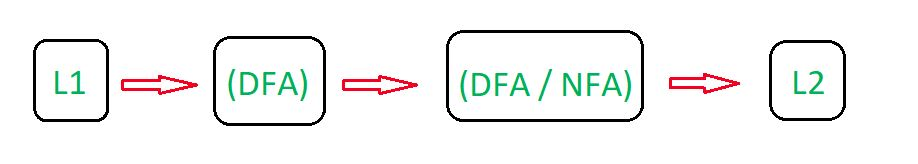Let’s understand the Reversal process in Deterministic Finite Automata (DFA) with the help of below example.

Example-1:
Designing a DFA for the set of string over {a, b} such that string of the language start symbol ‘a’. The desired language will be formed:

L1 = {a, aa, ab, aab, aaabb, aabab, .......}

In L1, each string have starting element a. State Transition Diagram for the language L1: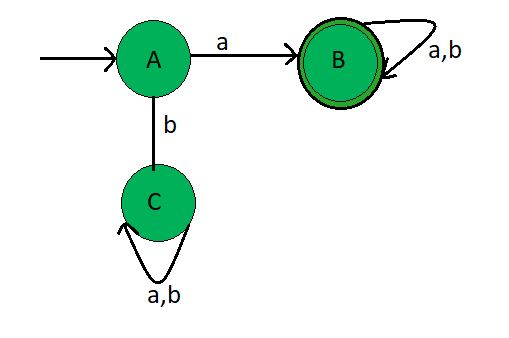This DFA accepts all the string starting with a. Here, State A and C are normal state but state B is final state. Now, Reversing the L1 language which gives the final result of the language L2.

State Transition Diagram of L2 (reverse of L1):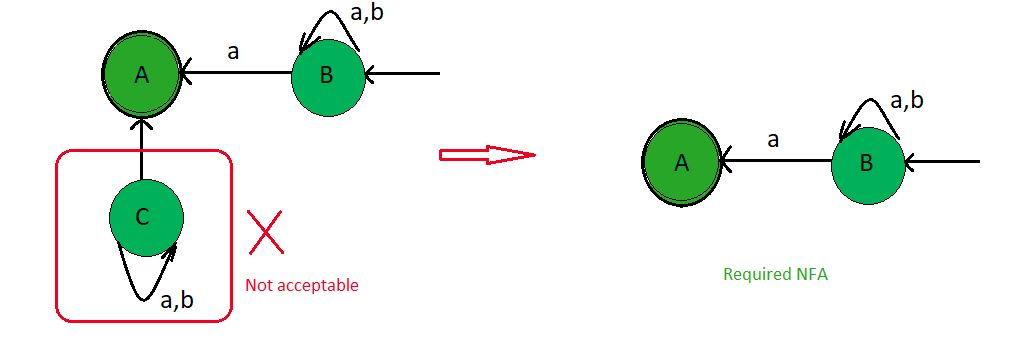Thus as we see that L2 have been obtained through reversal process and this final NFA accept all the language containing strings ending with a symbol ‘a’.

l = {a, ba, bba, ababa, ......}

Explanation:

• Same states (A, B, C) are draw as present in original state diagram
• Since A is the initial state make it as final state.
• Since B is the final state make it as initial state.
• Reversing the edges, since previously edges point A to B and A to C .Change the direction, now edges points toward B to A and C to A.
• Assign the values as same as original.
• Draw the loop as in original state diagram
• Since there is no incident edge on the state C so, we can reduce this state C.
• Since there is no transition for ‘a’ and ‘b’ in state A .Therefore resultant FA is NFA.

Example-2:
Designing a DFA for the set of string over {a, b} such that string of the language contain even number of’a’. The desired language will be formed:

L1 = {, aa, aab, aba, aab, aababa, .......}

In L1, each string having even number of element a.

State Transition Diagram for the language L1: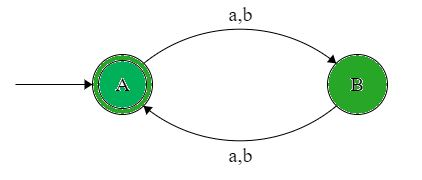This DFA accepts all the string containing even number of element a.Here, State A is initial state and state A is final state. Now, Reversing the L1 language which gives the final result of the language L2.

State Transition Diagram of L2 (reverse of L1):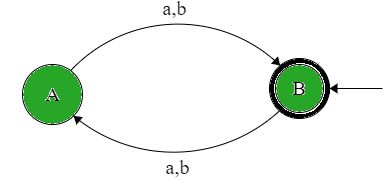Thus as we see that L2 have been obtained through reversal process and this final DFA accepts all the string containing even number of element a which is same as the original language.

L2 = {, aa, aab, aba, aab, aababa, .......}

Explanation:

• Same states (A, B) are draw as present in original state diagram.
• Since A is the initial state make it as final state.
• Since A is the final state make it as initial state.
• Reversing the edges, since previously edges point A to B and so on.Change the direction, now edges points toward B to A and so on.
• Assign the values as same as original.
• Since there is transition for ‘a’ and ‘b’ in state A and B.Therefore resultant FA is DFA.
• Since State diagram after and before reversal are same.Therefore L1 = L2

Attention reader! Don’t stop learning now.  Practice GATE exam well before the actual exam with the subject-wise and overall quizzes available in GATE Test Series Course.

Learn all GATE CS concepts with Free Live Classes on our youtube channel.

My Personal Notes arrow_drop_up